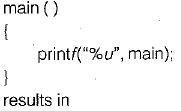Courses

# Programming In C++, C And Java (Basic Level) - 1

## 10 Questions MCQ Test Question Bank for GATE Computer Science Engineering | Programming In C++, C And Java (Basic Level) - 1

Description
This mock test of Programming In C++, C And Java (Basic Level) - 1 for Computer Science Engineering (CSE) helps you for every Computer Science Engineering (CSE) entrance exam. This contains 10 Multiple Choice Questions for Computer Science Engineering (CSE) Programming In C++, C And Java (Basic Level) - 1 (mcq) to study with solutions a complete question bank. The solved questions answers in this Programming In C++, C And Java (Basic Level) - 1 quiz give you a good mix of easy questions and tough questions. Computer Science Engineering (CSE) students definitely take this Programming In C++, C And Java (Basic Level) - 1 exercise for a better result in the exam. You can find other Programming In C++, C And Java (Basic Level) - 1 extra questions, long questions & short questions for Computer Science Engineering (CSE) on EduRev as well by searching above.
QUESTION: 1

### Choose the correct statements:

Solution:

Enum variable type is a special flavor of a long type variable with an enum, we can specify a number of valid values for that variable and descripted constant names for the values of the enum. These values are used instead of constant. Enum variable can be declared. The advantage of enum is it provide logical clarity of a program.

QUESTION: 2

### Consider the following statements: # define hypotenuse (a, b) sqrt (a*a + b*b); The macro-call hypotenuse (a + 2, b + 3);

Solution:

The macro call will be expanded as
sqrt (a + 2 * a + 2 + b + 3 * b + 3)
i.e. sqrt (3 * a + 4 * b + 5).

QUESTION: 3

### For the previous question, which of the following macro-calls, will find the hypotenuse of a right angled triangle with sides a + 1 and b + 1 ?

Solution:

Hypotenuse of right angles triangle (a+1), (b+1) will be
h = (a + 1)2 + (b + 1)2
= a2 + 2a + 1 + b2 + 2b + 1
= a2 + b2 + 2a + 2b + 2
which is not generated by any option.

QUESTION: 4

If p is pointer to an integer and t is a pointer to a character then size of (p) will be

Solution:

Pointer to an integer and pointer to a character are equivalent when the context of size of operator is used.

QUESTION: 5

1. Both are exactly same.
2. Array is a constant pointer.
3. Pointer is an one-dimensional array.
4. Pointer is a dynamic array.

Solution:

Array and pointer are same in implementation. By using pointer we can access any of the index array. Array is a constant pointer and pointer is a one dimensional dynamic array.

QUESTION: 6

Solution:

With macro no recursion is possible, pointers cannot be used with macro identifiers and no type checking will be done and it increase the code size. So, function will be advisable to used instead of macro.

QUESTION: 7

Use of macro instead of function is recommended

Solution:

Macro will reduce the execution time and can be used when there is a loop with a function call inside.

QUESTION: 8

The number of additions performed by the above program fragment is

Solution:

d = d + 1
= 9000 times
++z = 30 times
++j = 30 x 30 times
++K = 30 x 30 x 30 times
i + j + k = (30 x 30 x 30) x 2
So,
Total = 9000 + 900 + 30 + (27000 + 2 x 27000)
= 9930 + (3 x 27000)

QUESTION: 9

The following program:Solution:

Like array name, name of a function is a pointer to it. The given program results in starting address of function main (), which is pointer to it.

QUESTION: 10

Which of the following is false?

Solution:

Since address to some of the variable assigned at run time which is assigned by operating system. Two variable can have same address at different instance of time.